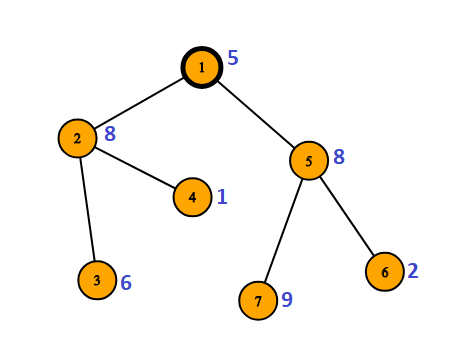Powerful Pair in Tree
Tag(s):

## Algorithms, Algorithms, Medium-Hard, Square Root Decomposition, Tree

Problem
Editorial
Analytics

Let’s define a term “Powerful Pair” as pair of two integer numbers, say A and B such that bitwise xor of these integers ( say, A xor B ) is a power of 2.

You are given a tree rooted at vertex 1 and total N vertices where each node contains a value in it. You have to answer Q queries. In each query, you will be given two vertices U and V, your task is to count the number of pairs of vertices (X, Y) (not necessarily distinct) such that X belongs to the subtree rooted at U and Y belongs to the subtree rooted at V and values in these vertices form a Power Pair.

Input:

Input starts with an integer N (1<=N<=100000), denoting the total number of nodes in tree. Next line contain N space separated integers denoting the values in node starting from 1 to N, which is nonnegative integer having value at most 100000. Next N-1 line contain two space separated integers, denoting an edge in tree. Next line will contain a single integer Q (1<=Q<=100000), denoting total number of quires. Following Q lines will contain two integers U and V separated by space between them.

Output:

For each query, output an integer denoting total number of ways of forming powerful pairing in between the values of subtrees given in each query.

SAMPLE INPUT
7
5 8 6 1 8 2 9
1 2
1 5
2 3
2 4
5 6
5 7
2
2 5
1 2

SAMPLE OUTPUT
3
4

ExplanationIn sample 1, there ate 3 powerful pairing in between subtree rooted at 2 and 5 , which are :

( 2 , 7 )
( 4 , 7 )
( 3 , 6 )

Time Limit: 8.0 sec(s) for each input file.
Memory Limit: 256 MB
Source Limit: 1024 KB
Marking Scheme: Marks are awarded when all the testcases pass.
Allowed Languages: Bash, C, C++, C++14, Clojure, C#, D, Erlang, F#, Go, Groovy, Haskell, Java, Java 8, JavaScript(Rhino), JavaScript(Node.js), Julia, Kotlin, Lisp, Lisp (SBCL), Lua, Objective-C, OCaml, Octave, Pascal, Perl, PHP, Python, Python 3, R(RScript), Racket, Ruby, Rust, Scala, Swift, Swift-4.1, TypeScript, Visual Basic

## CODE EDITOR

Initializing Code Editor...

## This Problem was Asked inChallenge Name

August Circuits '18

OTHER PROBLEMS OF THIS CHALLENGE
• Algorithms > Searching
• Algorithms > Dynamic Programming
• Algorithms > Sorting
• Algorithms > String Algorithms
• Basic Programming > Implementation

?